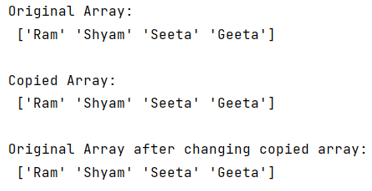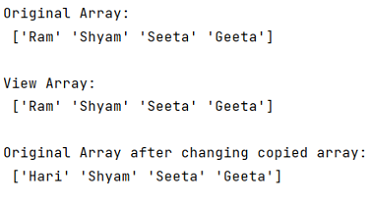# NumPy Array Copy vs View

Learn about the difference between NumPy array copy() and view() methods with examples. By Pranit Sharma Last updated : September 22, 2023

## NumPy array.copy() Vs. array.view() Method

NumPy is an abbreviated form of Numerical Python. It is used for different types of scientific operations in Python.

Numpy is a vast library in python which is used for almost every kind of scientific or mathematical operation. It is itself an array that is a collection of various methods and functions for processing the arrays.

There are a lot of methods included inside the NumPy library which are applicable on arrays (single dimension or multi-dimension). Two of the useful methods of NumPy array are copy() and view().

## Difference between copy() and view() methods

The difference between copy() and view() is not a complex concept to understand. When we use copy(), it makes a new copy of an array and any changes applied to the copied array will not make any impact on the original array. On the other hand, a view() is a representation of the original array where if any changes are made to the view, it will make an impact on the original array or vice-versa.

Let us understand the difference between copy() and view() methods with the help of an example,

## Example of NumPy array.copy() method

```# Importing numpy package
import numpy as np

# Creating an array
array = np.array(['Ram','Shyam','Seeta','Geeta'])

# Print array
print("Original Array:\n",array,"\n")

# Making a copy
copy = array.copy()

# Print copy
print("Copied Array:\n",copy,"\n")

# Making changes to copied array and
# printing original array
copy = 'Hari'

print("Original Array after changing copied array:\n",array)
```

### Output

The output of the above program is:As we can observe from the above example, making changes to the copy of the array, the original array is not affected.

## Example of NumPy array.view() method

```# Importing numpy package
import numpy as np

# Creating an array
array = np.array(['Ram','Shyam','Seeta','Geeta'])

# Print array
print("Original Array:\n",array,"\n")

# Making a view
view = array.view()

# Print view
print("View Array:\n",view,"\n")

# Making changes to copied array and
# printing original array
view = 'Hari'

print("Original Array after changing copied array:\n",array)
```

### Output

The output of the above program is:Also, we can observe that after making changes in view, the original array has also been changed.